• 多路归并排序
2021-06-01 11:26:39

使用python实现多(K)路归并外部排序，解决小内存排序大文件问题

上一篇中，我们实现了一般的归并排序 归并排序递归与非递归-Python实现

在实际工作中，多个有序数列合并成一个，大文件或多个大文件合并成一个并排序的需求常见并不少见，首先，先来看一下多个有序数列情况

## 合并多个有序数组

比如现在有四路：

• a0: [1, 3, 6, 7]
• a1: []
• a2: [3, 5, 7, 19]
• a3: [9, 12, 87, 98]

### 保存每路最小值

第一步需要知道每一路的最小值，如果每一路用数组表示的话需要保存对应的下标，并保存为min_map

• 第0路: 1
• 第1路: 没有值
• 第2路: 3
• 第3路: 9

初始的 min_map:

{0: (1, 0), 2: (3, 0), 3: (9, 0)}


### 获取最小值中的最小值

第二部需要将最小值取出来然，后检查被取出值的那一路是否还剩下。

其他元素如果存在，则修改min_map里面对应的值，如果不存在，则删除掉min_map里面对应的记录，以表示该路已经没有元素需要遍历了

代码：

#!/usr/bin/env python
# -*- coding: utf-8 -*-

# 多路归并: 将已经排序好的多个数组合并起来

def nw_merge(arrs):
"""
需要知道每一路的最小值
第0路: 1
第1路: 没有值
第2路: 3
第3路: 9
"""

result = []
min_map = {} # 用min_map 保存每一路的当前最小值
for inx, arr in enumerate(arrs):
if arr:
min_map[inx] = (arr, 0)

print("初始化的每一路最小值min_map", min_map)

while min_map:
"""
需要知道每一路的最小值里面哪一路的最小值, 以及最小值所在的那一路的index
"""

min_ = min(min_map.items(), key = lambda m: m)
way_num, (way_min_v, way_inx) = min_
result.append(way_min_v)

"""
检查被取出值的那一路是否还剩下其他元素， 如果存在， 则修改min_map里面对应的值， 如果不存在，
则删除掉min_map里面对应的记录， 以表示该路已经没有元素需要遍历了
"""
way_inx += 1
if way_inx < len(arrs[way_num]):
min_map[way_num] = (arrs[way_num][way_inx], way_inx)
else:
del min_map[way_num]
return result

a0 = [1, 3, 6, 7]
a1 = []
a2 = [3, 5, 7, 19]
a3 = [9, 12, 87, 98]
arrs = [a0, a1, a2, a3]

print("a0:", a0)
print("a1:", a1)
print("a2:", a2)
print("a3:", a3)

result = nw_merge(arrs)

print("最终合并的:", result)


### 输出

"""
a0: [1, 3, 6, 7]
a1: []
a2: [3, 5, 7, 19]
a3: [9, 12, 87, 98]

初始化的每一路最小值min_map {0: (1, 0), 2: (3, 0), 3: (9, 0)}
"""

# 最终合并的:
[1, 3, 3, 5, 6, 7, 7, 9, 12, 19, 87, 98]


## 对超大文件排序(10G的日志，512M的内存)

绕不开归并核心思想，分治，先拆成小文件，再排序，最后合并所有碎片文件成一个大文件

### 拆文件

首先第一步，大文件拆分成　x　个 block_size 大的小文件，每个小文件排好序


def save_file(l, fileno):
filepath = f"/home/xxx/{fileno}"

f = open(filepath, 'a')
for i in l:
f.write(f"{i}\n")
f.close()
return filepath

def split_file(file_path, block_size):
f = open(file_path, 'r')
fileno = 1
files = []
while True:
if not lines:
break
lines = [int(i.strip()) for i in lines]
lines.sort()
files.append(save_file(lines, fileno))
fileno += 1
return files


### 合并

将拆分成的小文件合并起来，然后将归并的东西写到大文件里面去，这里用到的是上面的多路归并的方法

def nw_merge(files):
fs = [open(file_) for file_ in files]
min_map = {}
out = open("/home/xxx/out", "a")
for f in fs:

while min_map:
min_ = min(min_map.items(), key = lambda x: x)
min_f, min_v = min_
out.write("{}".format(min_v))
out.write("\n")
if nextline:
min_map[min_f] = int(nextline.strip())
else:
del min_map[min_f]


### 全部代码

import os
from pathlib import Path

def nw_merge(files):
fs = [open(file_) for file_ in files]
min_map = {}  # 用来记录每一路当前最小值
out = open(Path(".") / "out/integration.txt", "a+")
for f in fs:

while min_map:  # 将最小值取出，　并将该最小值所在的那一路做对应的更新
min_ = min(min_map.items(), key=lambda x: x)
min_f, min_v = min_
out.write(f"{min_v}\n")
if nextline:
min_map[min_f] = int(nextline.strip())
else:
del min_map[min_f]
for f in fs:
f.close()
out.close()

def save_file(l, fileno):
path = Path(".") / "split"
filepath = path / f"{fileno}"
info = '\n'.join(map(str, l))
with open(filepath, "a+") as f:
f.write(f"{info}")

return filepath

def split_file(file_path, block_size):
fileno = 1  # 文件数
files = []  # 小文件目录
with open(file_path, 'r') as f:
while True:
if not lines:
break
lines = [int(i.strip()) for i in lines]  # 生成一个列表
lines.sort()  # 排序
files.append(save_file(lines, fileno))
fileno += 1
return files

if __name__ == "__main__":
# 每行单个数字
file_path = Path(".") / "tests.txt"
block_size = 500 * 1024 * 1024 # 500K
num_blocks = os.stat(file_path).st_size / block_size
files = split_file(file_path, block_size)
nw_merge(files)


阅读更多python 数据结构 归并排序 大文件
更多相关内容
• 然后捣鼓了一下，学到了一种新技能 - 多路归并排序 学习的过程是这样的： 第一步 ： 把存储着很多很多很多数的文件进行切割，切割成N个小文件，每个小文件都存储一些无序的数，具体切割的每个文件的大小不要超过...

雪压枝头低，虽低不着泥

今天准备放假，无聊看到一个场景题，问题如下：

有一个文件里面存储着很多很多很多的无序的数，然后要求进行一个排序，内存限定，磁盘足够

然后捣鼓了一下，学到了一种新技能 - 多路归并排序

学习的过程是这样的：

第一步 ：  把存储着很多很多很多数的文件进行切割，切割成N个小文件，每个小文件都存储一些无序的数，具体切割的每个文件的大小不要超过机器的内存，如下图：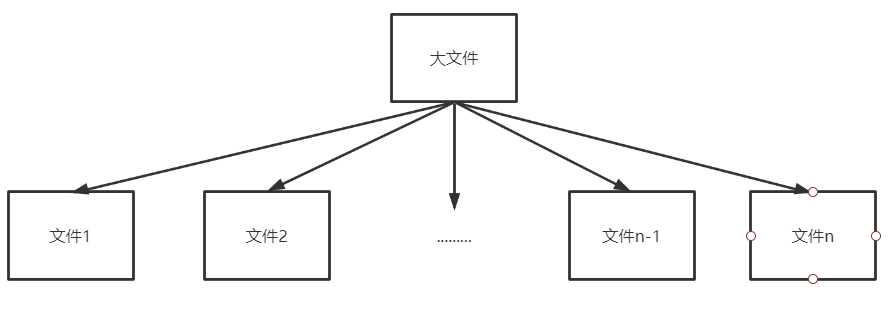第二步： 文件切割后标记每个文件唯一的标识，暂且标识为 文件1、文件2 .... 文件n； 然后对每个文件里面的数据进行内存排序，并把文件里最小的数记录到内存中，如下图；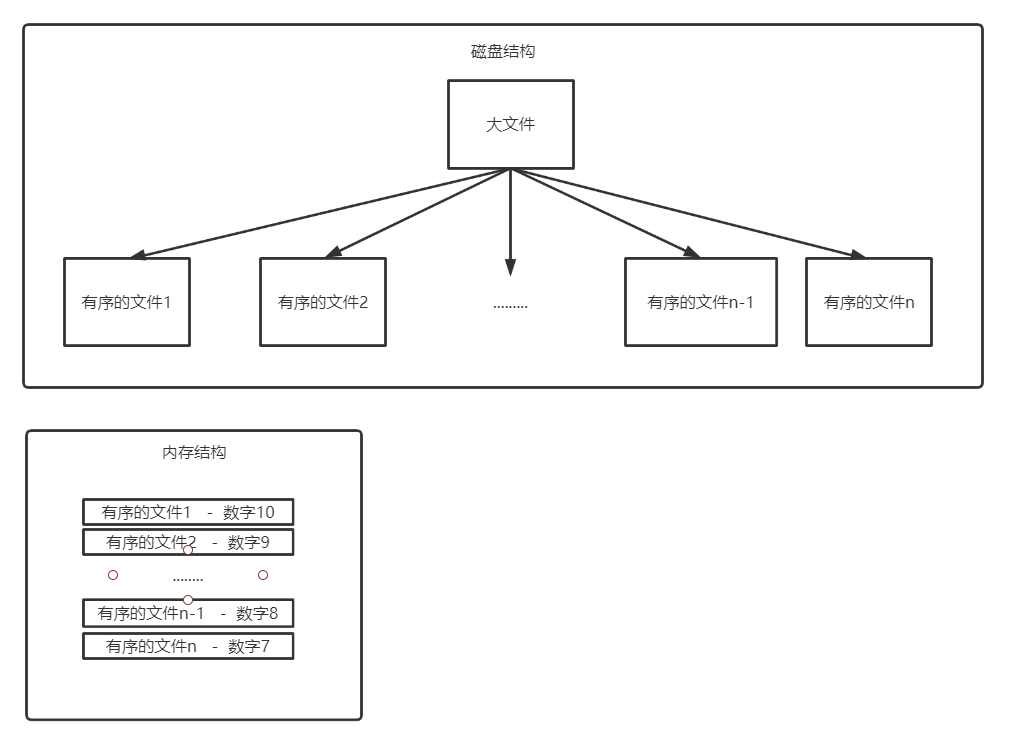第三步：磁盘创建一个新的文件，对内存里面的数字进行排序，将最小的数字追加到文件中，并记录最小的数字对应的文件，如这一轮中最小的数字是7，将7追加新文件，然后再从7对应的有序的文件n中取下一个数到内存结构的排序数组中，然后又再将最小的数字追加到新文件中.... 如此的反复，这样就可以保证内存只有一个数组在排序，相当于一个中转站，从磁盘取数排序，然后再将数追加到磁盘中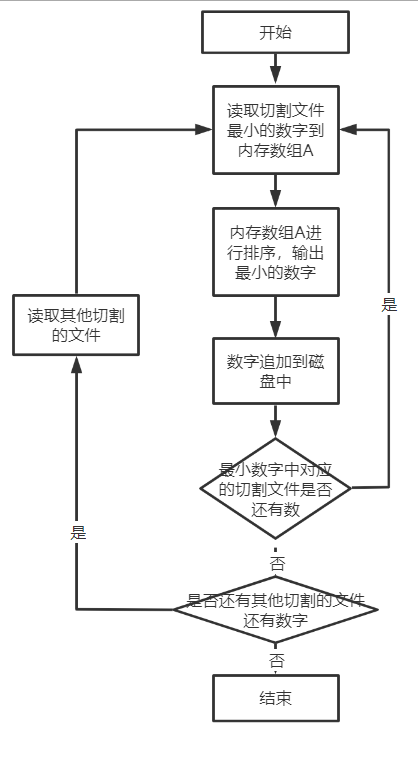最后，附上一个整体的数据流向图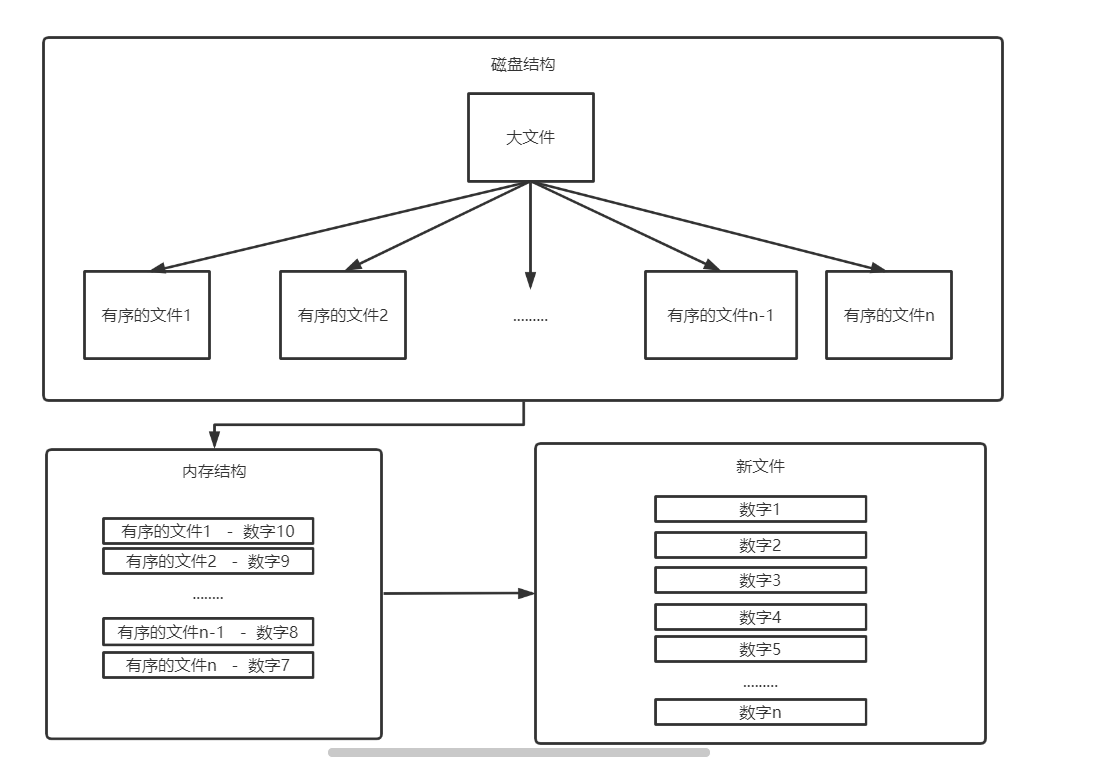展开全文java python
• 多路归并排序算法，主要针对于海量数据排序，代码中有注释。
• 其实归并排序挺好理解的，也挺好实现的。其实也挺像我们的平常分工合作的。就像一样事情分成几份，由不同的人去做。再合并起来，采用了分治的思想。 对于一个数列，也同是如此。 我们只需要不断地对着中点切分就...添加微信公众号可以索取资料
添加QQ群一起分享技术：895467044

啥也先不说，先上图

，上图最好理解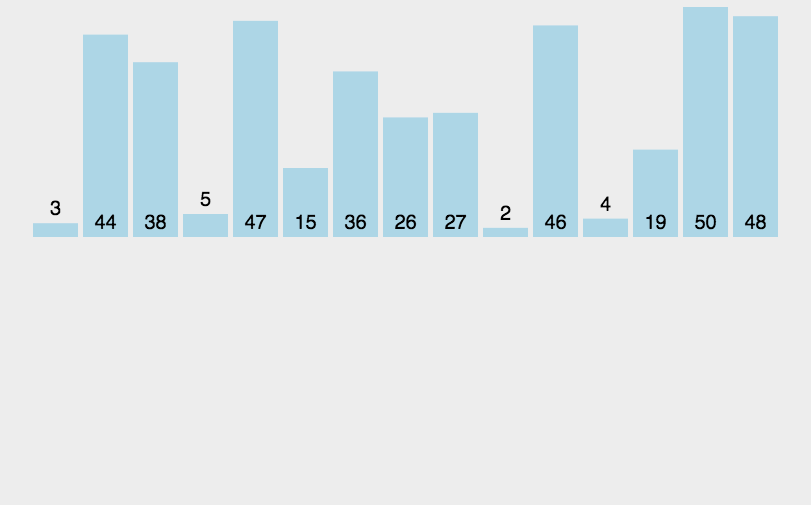其实归并排序挺好理解的，也挺好实现的。其实也挺像我们的平常分工合作的。就像一样事情分成几份，由不同的人去做。再合并起来，采用了分治的思想。

对于一个数列，也同是如此。

我们只需要不断地对着中点切分就可以了。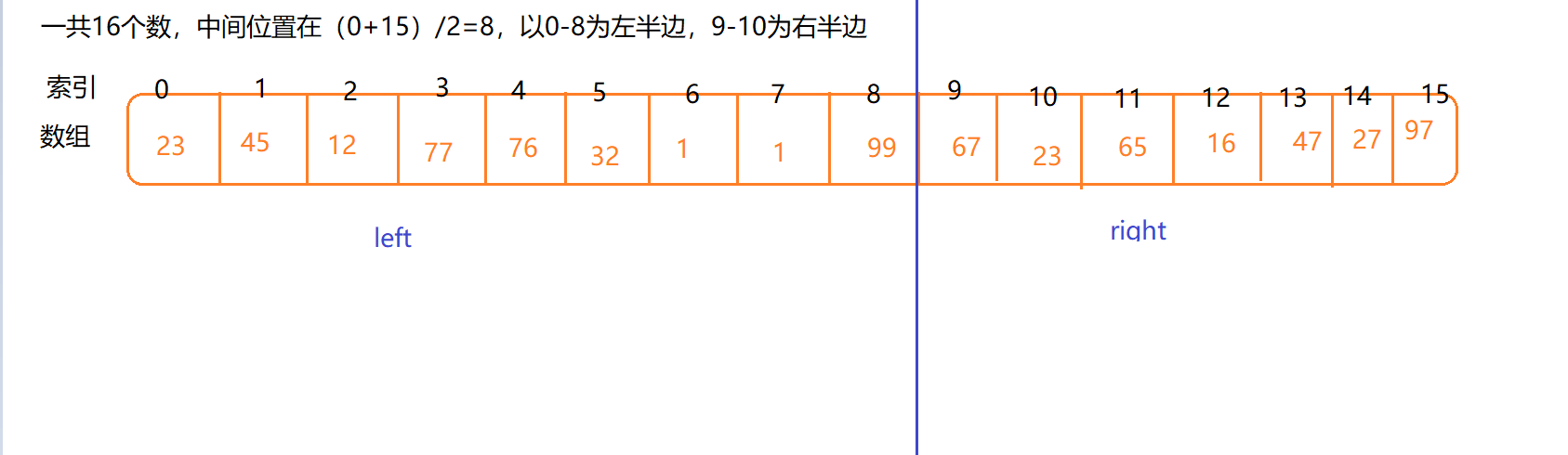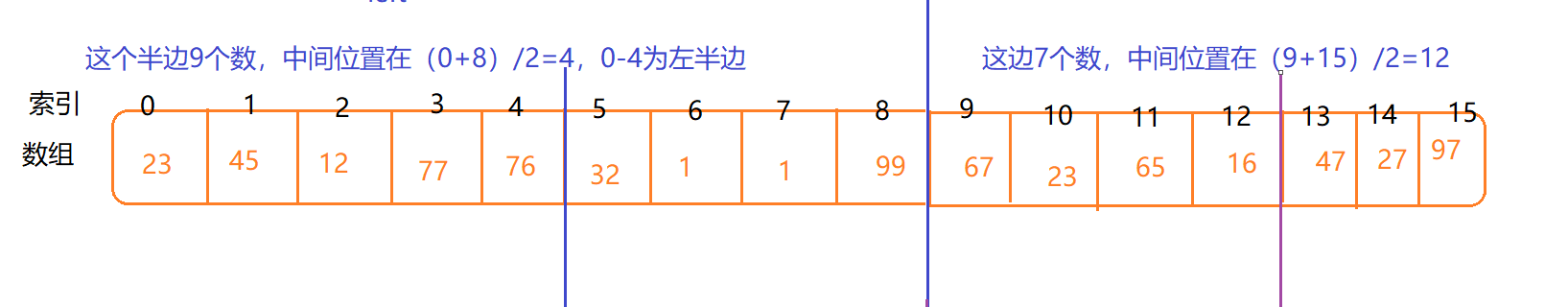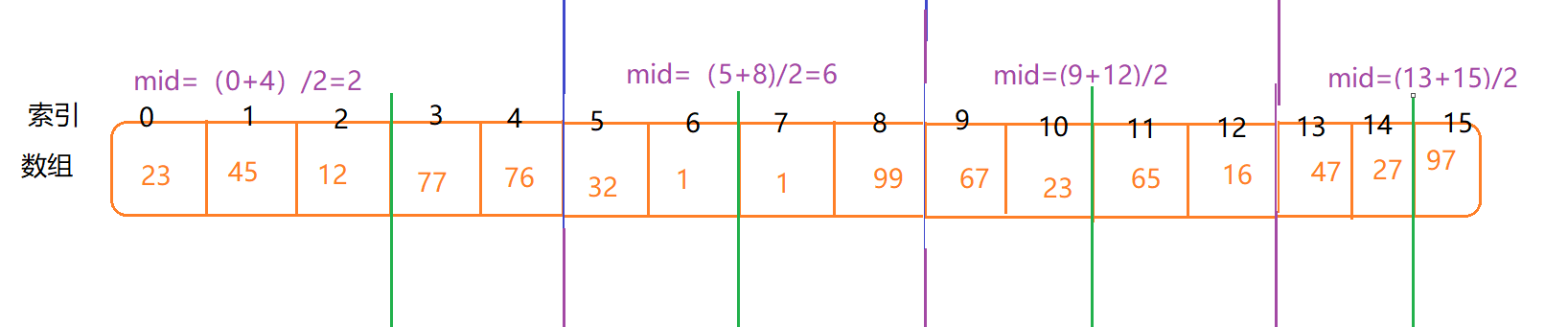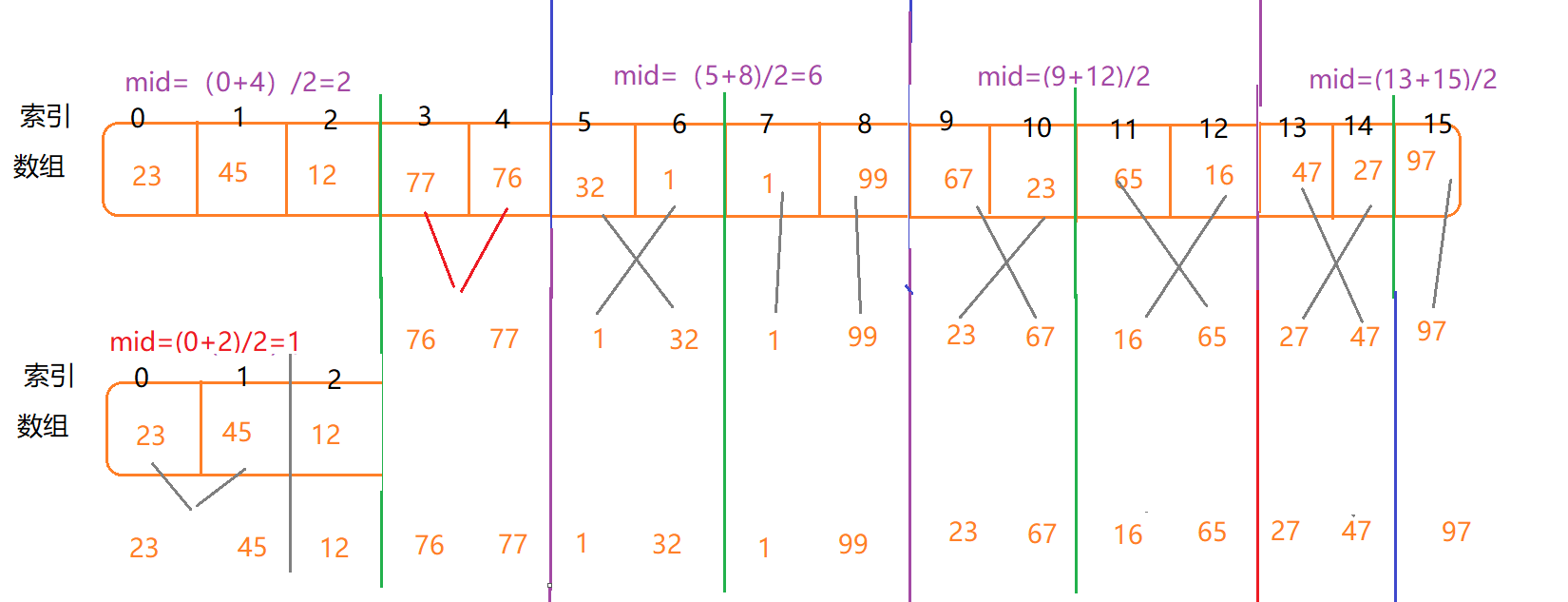就这样类似的下去，针对每一个每次分割的取键合并

总共可以分两个过程，一个cut，一个merge

cut：对数列进行分割。

merge：这个过程就是两个区间进行合并，可以新建要给数组，然后从两个已排序的数组依次比较，这样就可以将小的或者大的先放进新的数组。

具体可以看看代码实现：

public int[] sort(int[] nums,int start,int end) {
//如果只有一个节点了（start=end）没必要排序，直接将这个元素作为新数组返回就是了
if(nums==null||start==end) return new int[] {nums[start]};
//cut过程
//计算中点
int mid = (start+end)/2;
//这个包含了cut过程，就是将左半边和右半边分开
int[] left = sort(nums, start, mid);//计算左半部分，从start------mid
int[] right = sort(nums, mid+1, end);//计算右半部分，从mid+1------end

//merge过程
//新建一个数组，用来保存合并后的元素
int[] retu = new int[left.length+right.length];
//i是left的索引，j是right的索引，p是新数组索引
int i=0,j=0,p=0;

while(i<left.length&&j<right.length) {
//哪个小就先放入新数组，这是从小到大排序
if(left[i]<right[j]) {
retu[p]=left[i];
i++;
}else {
retu[p]=right[j];
j++;
}
p++;
}
//如果某一个数组比另一个数组长，那么它的索引肯定没有到最后，那么从它的索引到以后的全都是顺序的，那么直接顺序添加进去就行
if(i<left.length) for(;i<left.length;i++,p++) retu[p]=left[i];
else if(j<right.length) for(;j<right.length;j++,p++) retu[p]=right[j];
return retu;

}

当然这是一个数组的情况

前一天在leetcode发现了要给题目，是对链表进行排序，采用归并排序；

https://leetcode-cn.com/problems/sort-list/solution/sort-list-gui-bing-pai-xu-lian-biao-by-jyd/

可以去做做

public ListNode sortList(ListNode head) {
if (head == null || head.next == null)
//cut过程
//下面就是利用物理中的快慢速度，来找到中点
//一个是一次跳一个节点，另一个跳两个节点，同样的速度，不同的步长，当第二个到了终点，第一个还在正中心
ListNode fast = head.next, slow = head;
while (fast != null && fast.next != null) {//如果是偶数个的话（1234），fast==null；；；；；如果是奇数个的话(12345),fast.next=null
slow = slow.next;
fast = fast.next.next;
}
ListNode tmp = slow.next;//tmp保存后半部分链表的头节点
slow.next = null;//将前半部分与后半部分链表切开
ListNode left = sortList(head);//求的前半部分链表
ListNode right = sortList(tmp);//求的后半部分链表

//merge过程
ListNode h = new ListNode(0);//新的头节点，来讲left和right合并
ListNode res = h;
while (left != null && right != null) {
if (left.val < right.val) {
h.next = left;
left = left.next;
} else {
h.next = right;
right = right.next;
}
h = h.next;
}
h.next = left != null ? left : right;//将剩下的连接起来
return res.next;
}

这个链表的形式虽然找中心节点比较麻烦了，但是在每次新排序的节点中，空间开销不用新建整个数组，而是只需要头节点和一个索引指针（跟随新来的节点）就行。

所以对比上面两种，要知道归并排序的核心过程就是cutmerge

## 复杂度分析

关于时间复杂度很明显

我们一共要分为log n层

每一层，我们需要将每一个元素都放进一个新的数组里面去，也就是说每一层都会对所有的元素进行操作，复杂度n

总共就是nlog n

空间复杂度：

因为新建了一个数组，所以空间复杂度为O(n)，但是对于链表因为只需要一个头节点和索引指针，它的空间复杂度是常数级别的

# 归并排序思想使用

归并的思想主要用于外部排序

外部排序可分两步

• 待排序记录分批读入内存，用某种方法在内存排序，组成有序的子文件，再按某种策略存入外存。
• 子文件多路归并，成为较长有序子文件，再记入外存，如此反复，直到整个待排序文件有序。

外部排序可使用外存、磁带、磁盘，最初形成有序子文件长取决于内存所能提供排序区大小和最初排序策略，归并路数取决于所能提供排序的外部设备数。比如在mapreduce里面，就有个排序过程，当许多小文件溢写到磁盘时，多个小文件进行归并排序。

展开全文排序 归并排序 多路归并排序
• 归并排序左半边，归并排序右半边，合并 void merge_sort(vector<int> &nums,vector<int> reg,int start,int end){ if(start>=end) return ; int mid=(end-start)/2+start; int start1=start,...

# 1. 归并排序

base case：if(left>=right) return;
归并排序左半边，归并排序右半边，合并

void merge_sort(vector<int> &nums,vector<int> reg,int start,int end){
if(start>=end) return ;
int mid=(end-start)/2+start;
int start1=start,end1=mid;
int start2=mid+1,end2=end;
merge_sort(nums,reg,start1,end1);
merge_sort(nums,reg,start2,end2);//分治左、右半边
int t=start;
while(start1<=end1&&start2<=end2){
reg[t++]=nums[start1]>nums[start2]?nums[start1++]:nums[start2++];
}
while(start1<=end1)
reg[t++]=nums[start1++];
while(start2<=end2)
reg[t++]=nums[start2++];
while(start<=end)
nums[start]=reg[start++];//合并
}



# 2.k路归并排序、败者树

k路归并排序就是将路合并，可以通过增大k来减少外存的读写次数，从而减少归并时间，但是k增大，增大了比较的次数，通过败者树来确定k，从而不影响内部排序效率。

## 2.1败者树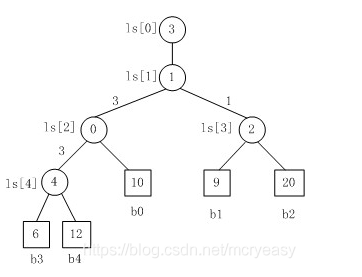如上所示，败者树是一颗完全二叉树，每个结点是一个选手，每个结点的父节点是比赛的结果的败者，数组b中的数据项发生了改变，需要重构败者树，重构的方式是将新进入选择树的结点与其父节点进行比赛，将败者存在父节点中，胜者再与上一级的父节点比赛，比赛沿着根节点的路径不断进行，直到ls处。把败者存放在ls处，胜者存放在ls处。

败者树的数据项发生变化：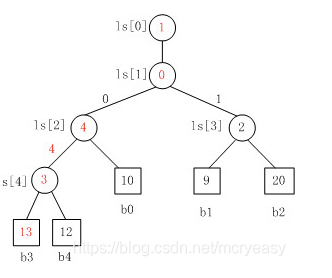对于败者树，数组的长度和原始数组的长度相等。
代码：数组b用来存储原始数组，数组ls用来生成败者树。ls存储的是b的索引，即子节点败者的索引
注释：数组b[s]的父节点是ls[t],t=(s+len)/2;
思想：b[s]与b[ls[t]]比较，败者存储在ls[t]中，s作为胜者继续向上比较。如果s败了，s和ls[t]交换作为胜者向上比较，如果s胜了，作为胜者向上比较。
ls[t]的父节点是ls[t/2]。
最终使得ls=s作为最终的胜者存储。
一言以蔽之：胜者不断和父节点比较。
过程：

1. 原始数据后面添加一个-1，作为初始化。
2. ls数组的长度和原始数组相同，初始值为-1所在的索引。
3. 从最后一个叶子节点（原始数据的最后一个元素）开始调整。
4. 调整的方式是逐步和父节点比较，失败就交换。最后得到胜者。
#include<iostream>
#include<vector>
using namespace std;
void swap(int& a,int& b){
int temp=a;
b=a;
a=temp;
}
void adjust(vector<int> &b,vector<int> &ls,int s,int len){
int t=(s+len)/2;
while(t>0){
if(b[s]>b[ls[t]]){
swap(s,ls[t]);
}
t/=2;
}
ls=s;
}
vector<int> creatls(vector<int>& nums){
int len=nums.size();
vector<int> b(len+1,-1);//最后一个元素-1是为了初始化，即-1永不败，也就是-1所在的索引永远不会出现在ls中
for(int i=0;i<len;i++)
b[i]=nums[i];
vector<int> ls(len,len);//ls的长度和原始数据的长度相同，初始值为-1所在的索引
for(int i=len-1;i>=0;i--){//从最后一个叶子结点调整败者树
}

return ls;
}
int main()
{
vector<int> b={10,9,20,6,12};
vector<int> ls=creatls(b);
for(int i=0;i<ls.size();i++)
cout<<ls[i];
}



## 2.2 归并排序

• 不断取出s=ls，表示第s个数组的元素是当前最小的，存入结果中
• 判断第s个数组是否遍历完，如果遍历完，存入INT_MAX，否则存入下一位到b[s]
终止条件：b[s]==INT_MAX，表示所有的数据遍历完了，结束。
#include<iostream>
#include<vector>
using namespace std;
#define MAX INT_MAX
void swaq(int& a,int& b){
int temp=a;
a=b;
b=temp;
}
void adjust(vector<int>& b,vector<int>& ls,int s,int k){
int t=(s+k)/2;
while(t>0){
if(b[s]>b[ls[t]])
swaq(s,ls[t]);
t/=2;
}
ls=s;
}
void creatls(vector<int>& b_,vector<int>& ls,int k){
vector<int> b(k+1,-1);
for(int i=0;i<b_.size();i++)
b[i]=b_[i];
for(int i=k-1;i>=0;i--)
}
void kmerge(vector<vector<int>>& nums,vector<int>& b,vector<int> &ls,int k){
vector<int> index(k,0);//index[i]表示第i个数组的下标
vector<int> res;
int s=ls;//s表示第几个数组
while(b[s]!=MAX){
for(int i=0;i<b.size();i++)
cout<<b[i]<<" " ;
cout<<endl;
res.push_back(nums[s][index[s]]);//按序到达
index[s]++;//第s个数组的下标
if(index[s]>=nums[s].size()){
b[s]=MAX;
}
else{
b[s]=nums[s][index[s]];
}
s=ls;

}
for(int i=0;i<res.size();i++)
cout<<res[i]<<" ";
}
int main(){
vector<vector<int>> nums{
vector<int>{1,3,6,9},
vector<int>{2,3,4,5},
vector<int>{3,5,5,6,12},
vector<int>{3,5,6,7},
vector<int>{3,4,6,6}
};
int k=5;
vector<int> b(k,0);
for(int i=0;i<b.size();i++)
b[i]=nums[i];
vector<int> ls(k,k);
creatls(b,ls,k);
kmerge(nums,b,ls,k);
return 0;
}



创建creatls是从最后一个节点调整到根节点
k路归并kmerge是不断取出最小的元素。注意：放入元素就要调整，整体结束条件和单独数组结束条件。

展开全文• 1 题目 这一种题目的描述，大概有以后两种： ...2 分割为小文件+多路归并排序 基本思路： step1：分割+排序 从头开始将大文件FileFileFile的一个小部分读入内存中，将这一小部分进行排序...大文件 多路归并 排序
• 文件归并排序 命令行说明： sort.py -i <input_filename> -o <output_filename> [-d ] [-c ] [-s ] [-t ] -i 输入源文件名 -o 输出目标文件名，如果未指定，则结果覆盖到源文件 -d 可选项，文件文本行的列分隔符，...
• 斯坦福大学那本数据库系统实现的一个实验，内容是查询执行这一章中的两阶段多路归并排序算法的C实现，全手工编写，希望对大家有所帮助~
• 数据库系统实现两阶段多路归并排序算法的C实现.docx
• 题目： 若外部存储上有3110400个...设归并趟数为s次，对n个记录进行排序，有m个归并段，要进行k路归并排序，则归并趟数s=log(k,m);(k为底数，m为真数 把u个记录分布在k个归并段上，调用merge算法进行归并得到每一...外部排序
• 多路归并的主要包括两个步骤，第一个步骤是对划分好的小数据块进行内部排序，第二步是归并所有的小数据块： 首先是内部排序，在此将1000000个数据分成十份，利用库函数qsort()进行排序，将排好序的子数据块分别...归并排序
• 磁盘记录 n 个 ...为此进行的是 (m/y)-1 路归并排序 x = (m/y)-1 如果在 m 路平衡归并排序过程中要求，输入、内部归并、输出三个处理同时进行的话，则需要设置 2m 个输入缓冲区和 2 个输出缓冲区 ...
• 归并排序(Merge)是建立在归并操作上的一种有效的排序算法。该算法是采用分治法（Divide and Conquer）的一个非常典型的应用，归并排序将两个已排序的表合并成一个表。分治思想：涉及递归，例如归并排序、堆排序、...
• 外部排序最常用的算法是多路归并排序，即将原文件分解成多个能够一次性装入内存的部分，分别把每一部分调入内存完成排序。然后，对已经排 序的子文件进行多路归并排序。 二、处理过程 （1）按可用内...
• 如果说语言的基础语法和业务逻辑编码的经验积累是术，那么数据结构与算法思想、设计模式就是道。就好像笑傲江湖里面华山派的剑宗、气宗一样，在最前期的时候剑宗的门人一般要比气...多路归并排序在大数据领域也是常...多路归并排序
• 外排序(磁盘排序)之多路归并排序的简单实现外排序(磁盘排序)之多路归并排序的简单实现
• 将文件中的数据关键字读取到内存中，完成多路归并排序（直接写入文件）。 实现如下：在VS2022中新建空项目，添加main.cpp文件。输入如下代码： #include <afx.h> #include <iostream> #include <...外排序 归并排序
• 本文面向和我一样的菜鸟233333，以通俗的形式讲述对于分批存储于文件中的大量数据进行多路归并排序的算法原理，并给出代码和详细的注释。忽略掉了实用性不强的复杂度证明部分。归并排序
•外部排序 数据结构
• 路归并模式：每次仅作两个文件的归并；当有个文件时，采用两两归并的模式，最终得到一个完整的记录文件。 二元归并树：二路归并模式的归并过程可以用一个二元树的形式描述，称之为二元归并树。 贪心求解： 任意...
• 绝大多数归并算法是每次n/2分，然后再合并排序。而本算法是将n维数组每次分为根号n后递归后归并排序，思想和二路归并类似，不同！
• 排序算法中的归并排序(Merge Sort)是利用”归并”技术来进行排序。归并是指将若干个已排序的子文件合并成一个有序的文件。 一、实现原理： 1、算法基本思路 设两个有序的子文件(相当于输入堆)放在同一向量中相邻的...
• 本文实例为大家分享了C语言实现归并排序的具体代码，供大家参考，具体内容如下 归并排序的基本思想： 将两个及其以上的有序表合并为一张有序表，把待排序序列通过分治法分为若干个有序子序列，然后每两个子序列合并...
• 数据库系统实现中二阶段多路归并排序的实现 C代码 生成文件时需要很长时间 仅仅是测试代码
• 先来看内部排序中最简单的2路归并排序算法。 算法核心操作是将一维数组中前后相邻的两个有序序列归并为一个有序序列，给定数组中序列界限i、m、n，用2个下标变量分别从i和j=m+1开始逐个往后处......